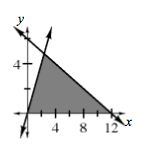### Home > INT3 > Chapter 2 > Lesson 2.2.5 > Problem2-129

2-129.

Graph these two lines on the same set of axes: $y=2x$ and $y=−\frac{1}{2}x+6$.

1. What are the $x$- and $y$-intercepts for each equation?

The $y$-intercepts are $(0,0)$ and $(0,6)$, and the $x$-intercepts are $(0,0)$ and $(12,0)$.

2. Shade the region bounded by the two lines and the $x$-axis.3. What are the domain and range of the region? How did you determine these values?

Look at the graph or the $x$-intercepts to find the domain. Find the point of intersection to determine the range.

The domain is $0\le x\le12$, and the range is $0\le y\le4.8$.